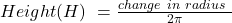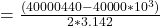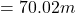## Consider a 40,000 km steel pipe in the shape of a ring that fits snuggly all around the circumference of the Earth. We are heating now the r

Question

Consider a 40,000 km steel pipe in the shape of a ring that fits snuggly all around the circumference of the Earth. We are heating now the ring so its temperature increased by 1 degree C. Now, the pipe will no longer be snug. How high the ring will now stand above ground level? (Make as many simplifications as necessary). Data: Coefficient of linear expansion for steel is 11*10-6 /degree C. This means, for example, that a 1-meter bar of steel that increases its temperature by 1 degree C will expand 11*10-6 meters (11 micrometers)

in progress 0
6 months 2021-08-25T12:22:18+00:00 1 Answers 5 views 0

The Height is  H = 70.02 m

Explanation:

We are given that the

Initial length is  =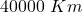=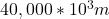from what we are told in the question the circumference of the circle is =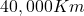This means that the Radius would be :

Let C denote the circumference

So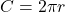=>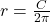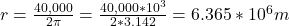We are told that 1-meter bar of steel that increases its temperature by 1 degree C will expand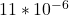meters

Hence

The final length would be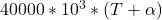Where T is the change in  temperature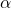is the Coefficient of linear expansion for steel

let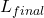denote the final length

So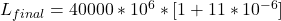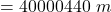Now the Height is mathematically represented as